Learn how to use R to visualize metrics in Wavefront.

The Wavefront service can handle your real-time, high-frequency data—fast ingestion, fast querying, fast analytics, visualization, and alerting. Wavefront Query Language can perform most of the transformations you need for daily monitoring. However, in some cases when you might want to perform computations that the query language doesn’t currently offer, or leverage a set of libraries you’ve already written in R to do analytics. In these cases, you can run R as a separate analytics layer on top of your Wavefront account.

With R+Wavefront, you can do just about any analysis or visualization you can imagine. Want to see a histogram of your metric at an arbitrary bin width? Or a heat map of the correlations between your metrics? Model your metrics for trends, seasonality, noise, and make a forecast about future behavior? Here’s how you learn how to do it.

## Prerequisites

To use R and Wavefront together, you need:

• A Wavefront account.
• A valid Wavefront token.
• A version of R at least 3.5.1 or higher.
• Optionally an R IDE, such as R Studio. Screenshots in this document were taken with RStudio running R 3.5.1.

## Installation

After setting up your Wavefront account and R environment, install the R packages that we’ll use in our demo, as well as the two (RCurl and rjson) used by the Wavefront library.

1. Within R, from the R prompt, run the following commands:

``````install.packages("dplyr")
install.packages("ggplot2")
install.packages("reshape2")
install.packages("scales")
install.packages("TSA")
install.packages("RCurl")
install.packages("rjson")
install.packages("httr")
``````
2. You may be asked to restart R during the installation; feel free to do so. Load the libraries into your R workspace:

``````library(ggplot2)
library(reshape2)
library(scales)
library(TSA)
library(dplyr)
library(RCurl)
library(rjson)
library(httr)
``````
3. Pull in the Wavefront R library:

``````source("http://wavefront-customer.s3.amazonaws.com/wavefront-2.5.R")
``````

This is an older version of this library:

``````source("http://wavefront-customer.s3.amazonaws.com/wavefront-1.7.R")
``````

A set of new functions appears in your IDE, all starting with `wf`. These are the functions that you will use to get data from Wavefront into R.

4. Enter the Wavefront server URL and your API token:

``````base <- "<wavefront_instance>"
token <- "<wavefront_api_token>"
``````

If you don’t have a token yet, see Generating an API Token.

## Getting Data Into R

The wavefront.R library allows you to perform the exact same queries through R that you would normally perform in the source field of the Wavefront chart, along with the same control over the time range. For example, you might normally enter `ts(requests.latency)` to grab the metric `requests.latency` over your 20 hosts: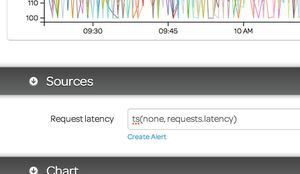In wavefront.R, you enter that query expression verbatim to retrieve the same data in numerical format, as an R data frame:

``````d <- wfquery(base, token, wfnow() - wfhours(2), wfnow() - wfminutes(1), 'ts(requests.latency)', clab='h')
``````

Let’s look at this R query in more detail. The base and token variables were set in the prerequisites, and allow `wavefront.R` to know where to query and how to authenticate that query. The third and fourth fields are the start and end times for the query, in epoch second format. The fifth field is the actual `ts()` query, and the final field is an option that tells the query to set the column labels (“clab” for short) of the resulting data frame to the h(ost) for that time series. Schematically, the function is:

``````<dataFrame> <- wfquery(serverURL, wavefrontAccountToken, startTime, endTime, query)
``````

The `wfnow()` function is a convenience function for the most recent time, so the range that we’re requesting is from `wfnow() - wfhours(2)`, or two hours ago, to `wfnow() - wfminutes(1)`, or one minute ago. This should give us exactly 120 observations (one per minute) over however many hosts were emitting the `requests.latency` metric. Let’s look at the result now. The data frame returned has one column per returned time series, as well as an initial column named `time`, which contains the epoch seconds for that row. For example, in the call above, the data frame has 120 (time) observations of 21 (time+host) variables; here’s the top of that data frame (viewed in RStudio):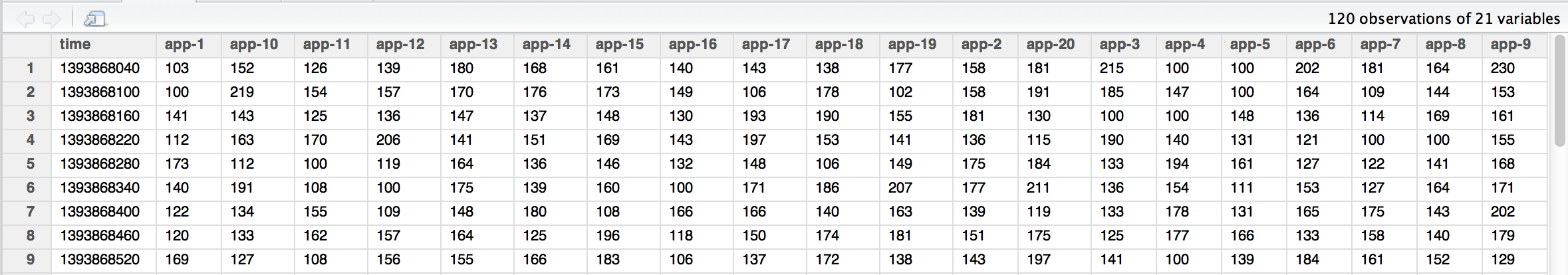This data frame can be manipulated like any other within R. If you wanted to see the request latencies divided by 100, or an average across all of the hosts, or the data from a month ago, you would do so in exactly the same way as you do from the Wavefront web site:

``````d <- wfquery(base, token, wfnow() - wfhours(2), wfnow() - wfminutes(1), 'ts(requests.latency) / 100', clab='h')
d <- wfquery(base, token, wfnow() - wfhours(2), wfnow() - wfminutes(1), 'avg(ts(requests.latency))', clab='h')
d <- wfquery(base, token, wfnow() - wfhours(2), wfnow() - wfminutes(1), 'lag("one month ago", avg(ts(requests.latency)))', clab='h')
``````

Note that query fields are surrounded by single quotes (‘’) while internal strings (such as the first argument to `lag`) are surrounded by double quotes (“”). You can even divide metrics by each other and then perform functions (like `lag`) on the result:

``````d <- wfquery(base, token, wfnow() - wfhours(2), wfnow() - wfminutes(1), 'lag("one month ago", ts(requests.failures.num) / ts(requests.total.num))', clab='h')
``````

All queries above look at the most recent 2 hour period of data, taken at the minute granularity. You can look at longer periods of data with coarser granularity. For example, here’s the most recent week of data, taken at the hour granularity `(granularity='h'`):

``````d <- wfquery(base, token, wfnow() - wfdays(7), wfnow(), 'ts(requests.latency)', clab='h', granularity='h')
``````

The resulting data frame has 169 observations (1 for each hour over the last week) over the 21 variables (1 time column, and 20 host columns):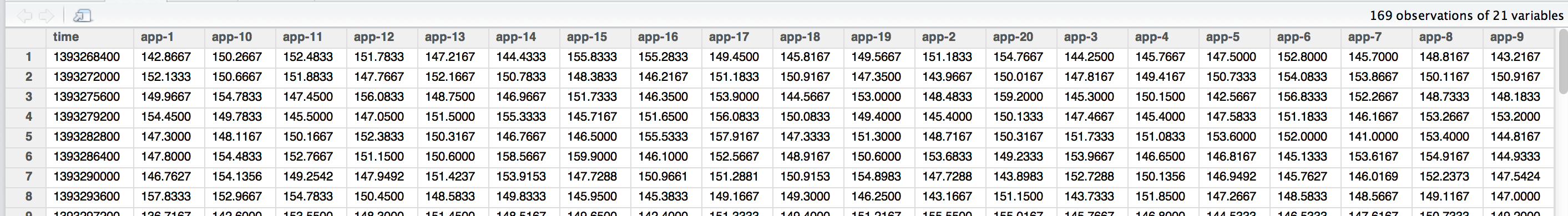The time rows are now spaced by 3600 seconds rather than 60 seconds. If you pick a long time range and a short granularity, the request eventually time out with no data returned. For most use cases either using a coarser granularity or using `lag()` on top of a short window fulfills your needs.

## Visualizing Data in R

Now that you’ve gotten your Wavefront data into R, you can do some interesting visualizations on it. First run a query against Wavefront to pull some data into a data frame:

``````# Grab requests.latency over the last 2 hours
d <- wfquery(base, token, wfnow() - wfhours(2), wfnow() - wfminutes(1), 'ts(requests.latency)', clab='h')
# Remove any missing data
d <- na.omit(d)
``````

### Histograms

``````# Show a histogram
ggplot(d, aes(d\$"app-1")) + geom_histogram(aes(y = ..density.., fill = ..count..), binwidth=5) + geom_density()
``````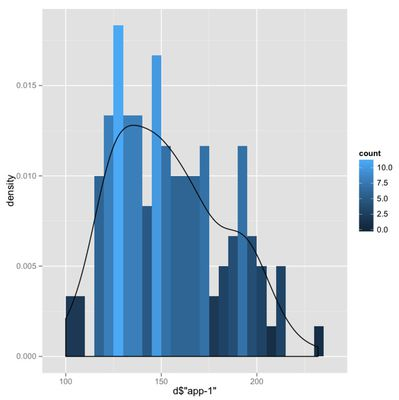### Heat Maps: Correlation Matrix

``````# Show a heat map of cross-correlations of all the app servers over the full 2h window
qplot(d\$"app-1", d\$"app-2") + geom_point(color="red", size=3)
cord <- cor(d)
for (index in 1:length(d)) cord[index,index] <- 0
d.m <- melt(cord)
ggplot(d.m, aes(Var1, Var2)) + geom_tile(aes(fill=value), colour="white") + scale_fill_gradient(low="white", high="steelblue")
``````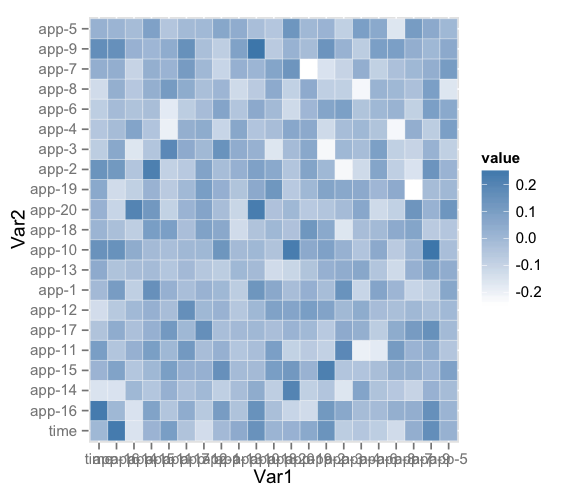## Analyzing Data in R

Beyond visualizing data, you can perform more complicated analysis on the data than is possible within the Wavefront Query Language. Here is a linear regression example: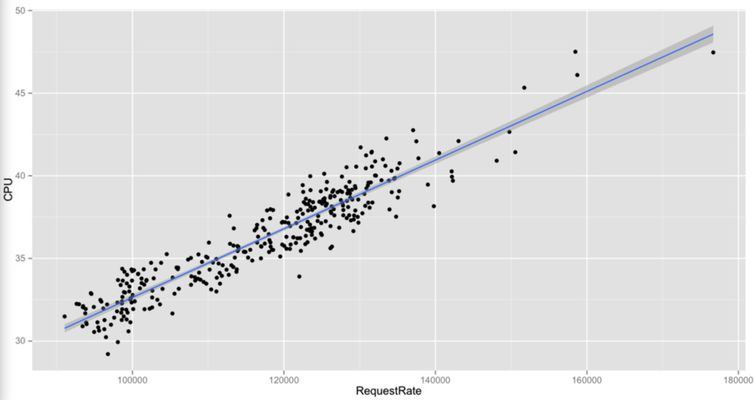### Example

``````queries <- c('ts(mem.used.percentage,source=app-1)','ts(cpu.loadavg.1m,source=app-1)')
dataset = wfqueryvl(base,token, wfnow() - wfhours(2), wfnow() - wfminutes(1),queries) # data frame containing data from both queries along with timestamp
scatterdata <- data.frame(Mem = dataset[],Cpu = dataset[]) # only metric values from both queries mapped based on timestamp
ggplot(scatterdata,aes(x=Mem,y=Cpu)) + geom_point(shape=19) +geom_smooth(method=lm)
``````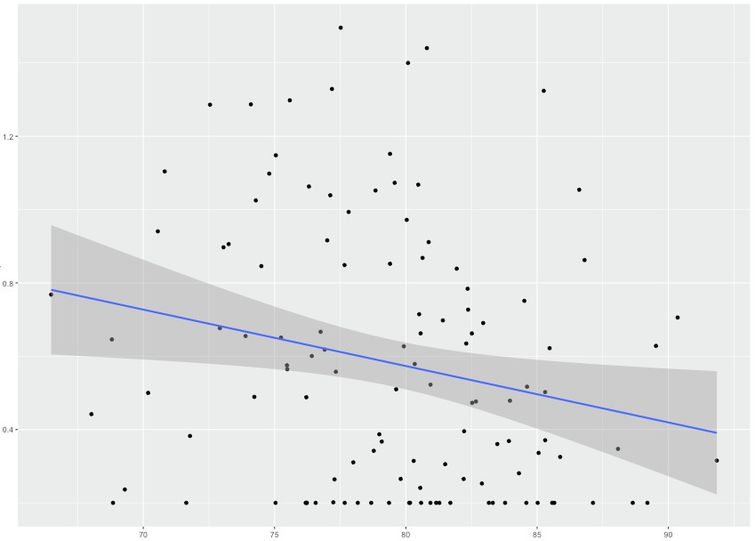## Resources

If you’re just getting started with R, there are a few resources to help you out. You don’t need to read any of these to follow along with this document, but they will help you understand what’s going on under the hood.

• Introducting R, Princeton: Very gentle introduction to the basic workspace, broken into a few easy-to-digest sections
• An Introduction to R, CRAN: A longer tutorial for R in terms of syntax and basic analysis/visualization
• The R Book, Michael Cawley: The best overall view of R as a mathematical/statistical tool, though at 1000+ pages, more of a reference than a tutorial.